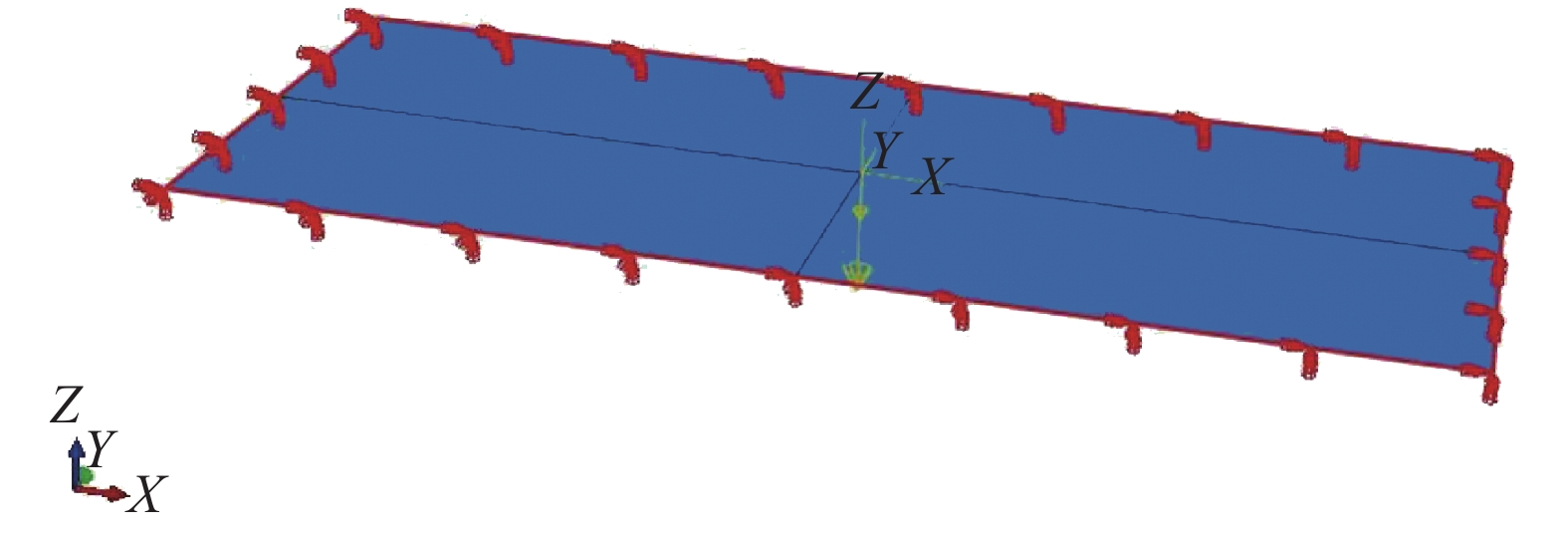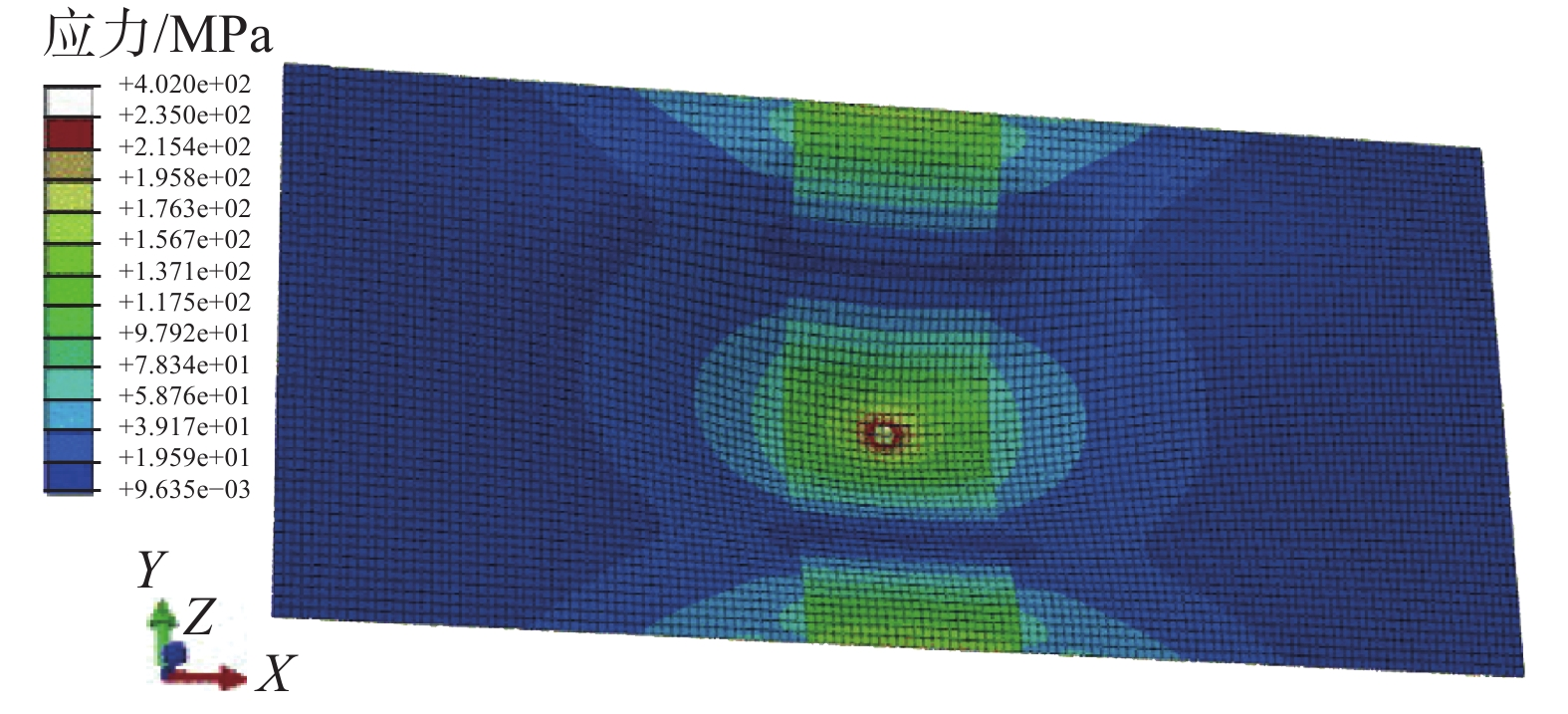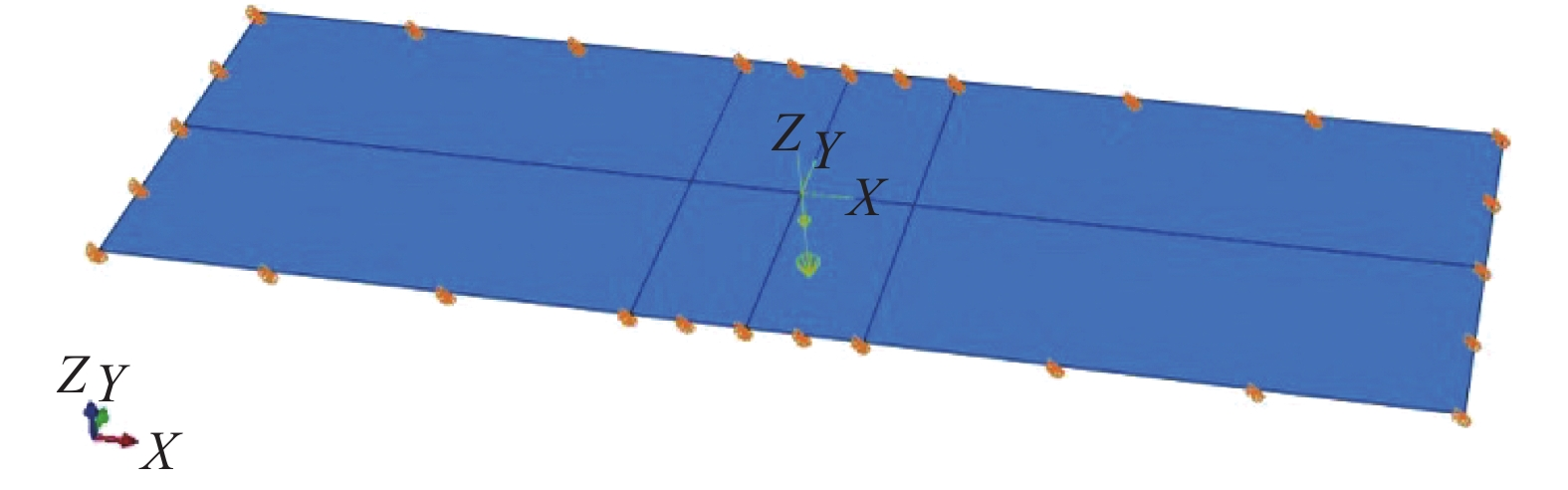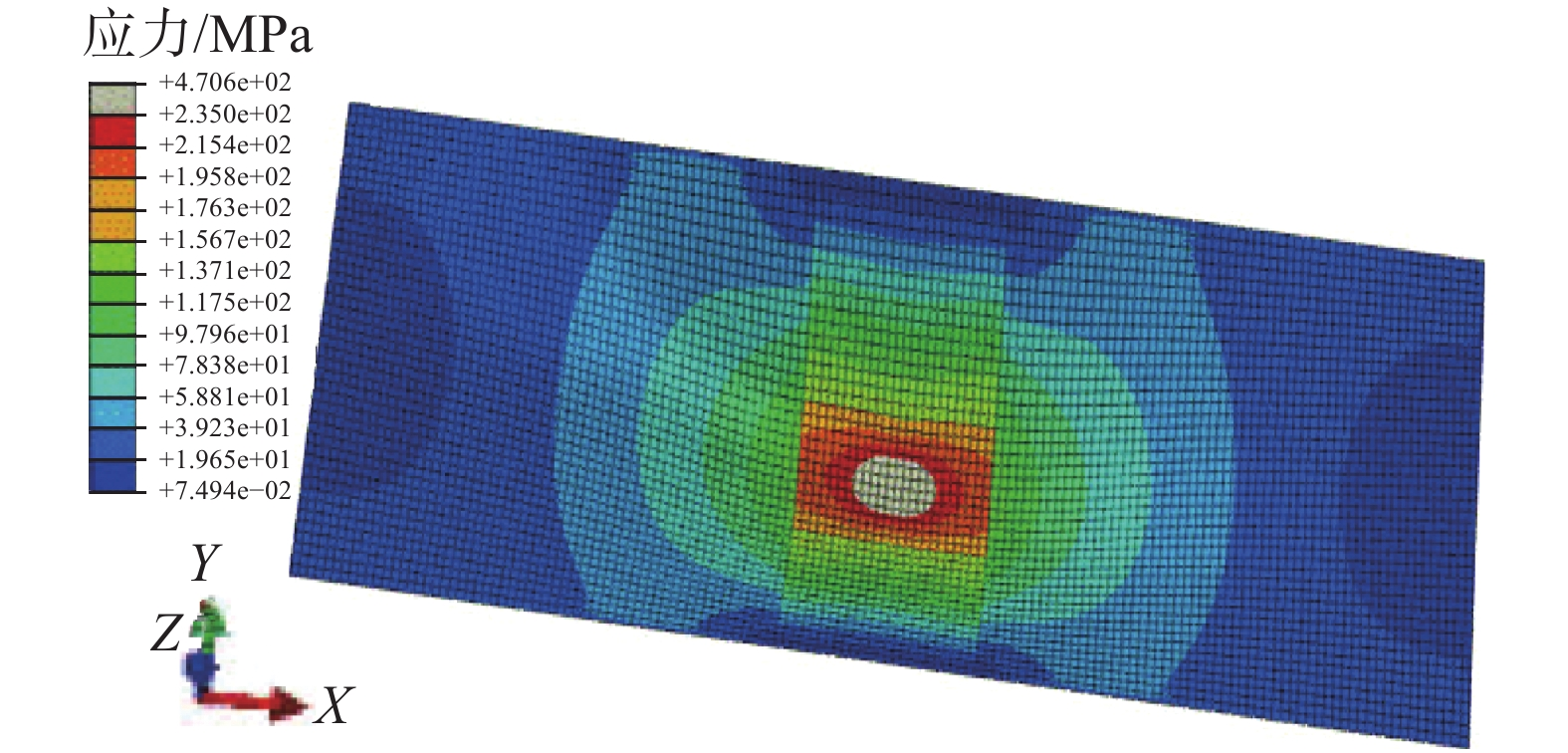﻿ 海洋平台铁舾装减重研究
«上一篇文章快速检索 高级检索

 应用科技2020, Vol. 47Issue (3): 6-10  DOI: 10.11991/yykj.2019080050

### 引用本文XIA Guangyin, DU Zhifu. A study of weight control over steel outfitting in offshore platform[J]. Applied Science and Technology, 2020, 47(3): 6-10. DOI: 10.11991/yykj.201908005.### 文章历史

A study of weight control over steel outfitting in offshore platform
XIA Guangyin, DU Zhifu
CIMC Offshore Engineering Institute Company Limited, Yantai 264670, China
Abstract: In general, the weight of the steel outfitting is ignored in the weight control of offshore platform, and the steel outfitting design is based on the experience of the engineers. However, controlling the steel outfitting weight is very important for the offshore platform. In this paper, a typical checkered plate design and weight control will be studied. The purpose is got a method for the steel outfitting design that is easy to operate and meet relevant codes. First of all, study relevant technical codes to ascertain the requirement for the design of steel outfitting. After that, research the design of the steel outfitting based on the codes. The research shows that it is very conservative in the traditional steel outfitting design based on experience. The steel outfitting data for selection in the design are given after exact calculation in this paper.
Keywords: offshore platform    weight control    design in terms of code    checkered plate    finite element method (FEM) check    normal stress    bending moment

1 规范设计分析研究

1.1 花钢板的规范计算依据

1.1.1 美国船级社设计依据

 ${\rm{}}t = 3s\sqrt h + 1\;{\rm{mm}}$ (1)

 $s=(t-1)/\left(3\sqrt{h}\right)\;\;{\rm{m}}$ (2)

 ${M}_{\max}=Ql/8$
 ${F}_{\max}=0.5Q$

 ${M}_{\max}=Ql/12$
 ${F}_{\max}=0.5Q$

 $t=3s\sqrt{1.5h}+1\;{\rm{mm}}$ (3)

 $s=(t-1)/\left(3\sqrt{1.5h}\right)\;{\rm{m}}$ (4)

 $\mathrm{S}\mathrm{M}=3.5sh{l}^{2}\;{{\rm{cm}}^{3}}$表 1 不同情况下的型材规格

1.1.2 中国船级社设计依据

 $t=3s\sqrt{Kh}\;{\rm{mm}}$ (5)

 $W=5sKh{l}^{2}\;{{\rm{cm}}^{3}}$表 2 按CCS规范计算不同情况下的型材规格
1.1.3 挪威−劳氏船级社设计依据

 $t = \frac{{15.8{k_a}s\sqrt {{p_d}} }}{{\sqrt {{\sigma _{{\rm{pd}}1}}{k_{{\rm{pp}}}}} }}\;{\rm{mm}}$ (6)

 $s = \frac{{t\sqrt {{\sigma _{{\rm{pd}}1}}{k_{{\rm{pp}}}}} }}{{15.8{k_a}\sqrt {{p_d}} }}\;{\rm{m}}$ (7)

 ${Z}_{s}=\frac{1\;000{l}^{2}s{p}_{d}}{{k}_{m}{\sigma }_{\mathrm{p}\mathrm{d}2}{k}_{\mathrm{p}\mathrm{s}}}\;{{\rm{cm}}}^{3}$表 3 按DNVGL规范计算不同情况下的型材规格

1.2 花钢板的力学计算依据

 ${\sigma }_{\max}=\frac{1\;000\alpha P}{{t}^{2}}\;{\rm{MPa}}$ (8)
 ${\omega }_{\max}=\beta {\left(\frac{1\;000b}{t}\right)}^{2}\frac{1\;000P}{Et}\;{\rm{mm}}$ (9)表 4 焊接花钢板最大正应力和最大挠度表 5 螺栓连接的花钢板最大正应力和最大挠度

2 有限元校核

1)工况1。板厚4.5 mm，焊接，取一个板长3.5 m、宽1.03 m的板格，按四边固支计算。有限元模型如图1所示。Download: 图 1 工况1有限元模型Download: 图 2 工况1应力云图

2)工况2。板厚4.5 mm，螺栓连接，取一个板长3.5 m、宽0.84 m的板格，按四边简支计算。有限元模型如图3所示。Download: 图 3 工况2有限元模型Download: 图 4 工况2应力云图

3 花钢板设计标准表 6 花钢设计选型表
4 结论

1)根据铁舾装从业技术人员的特点，制定出标准化的设计模板，简单明了，不需要复杂的计算就可以直接掌握。

2)从材料力学的基础上，依据实际使用情况，参照各大船级社规范，如ABS、DNVGL、CCS等，又不照搬规范，从理论上给出设计依据。

  ABS. Rules for building and classing mobile offshore drilling units[S]. 2017: 16, 84-85. (0)  夏广印, 徐勤花. 结构规范计算公式与经典力学理论的比较[J]. 船舶标准化工程师, 2016, 49(2): 10-14, 43. (0)  陈可越. 船舶设计实用手册——结构分册[M]. 北京: 中国交通科技出版社, 2007: 5-21. (0)  CCS. 海上移动平台入级规范[S]. 北京: 中国船级社, 2016: 32-44. (0)  DNVGL. DNVGL-OS-C101, Design of offshore steel structures, general- LRFD method[S]. 2018: 26-55. (0)  成大先, 机械设计手册[M]. 北京: 化学工业主版社, 2007: 162-163. (0)  中国机械工程学会, 中国机械设计大典编委会. 中国机械设计大典[M]. 南昌: 江西科学技术出版社, 2002: 101-102. (0)